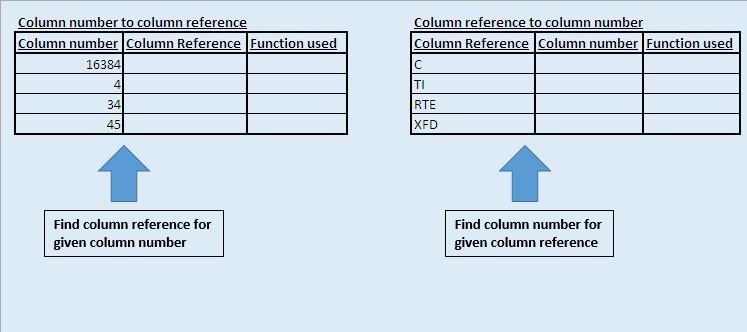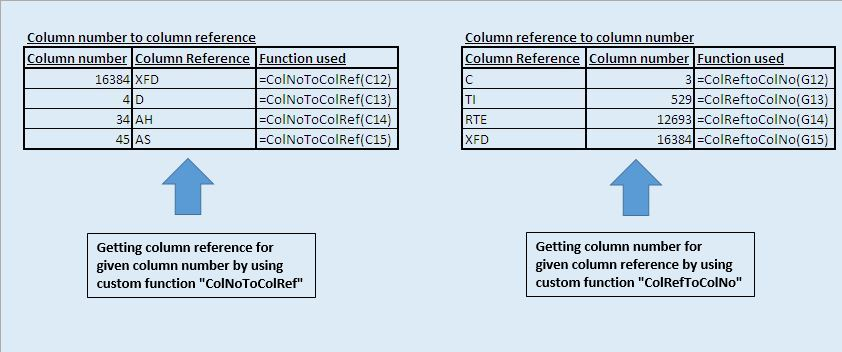# Conversion between column numbers and column references using VBA in Microsoft Excel

In this article, we will create two custom functions, one function to convert column numbers to column references and other function to convert column references to column numbers.

Raw data for this example consists of two sample data, one sample data contains random column numbers and the second sample data contains random column references.We have created two custom functions “ColNoToColRef” and “ColRefToColNo”. “ColNoToColRef” function is used to convert column numbers to column references. It takes integer values as input. Similarly, “ColRefToColNo” function is used to convert column references to column numbers.Code explanation

Above code is used to get the address of the specified cell.

Left(ColNoToColRef, InStr(1, ColNoToColRef, "\$") - 1)

Above code is used to extract the character to the left side of the symbol “\$”.

```
Option Explicit

Function ColNoToColRef(ColNo As Long) As String

If ColNo < 1 Or ColNo > Columns.Count Then

ColNoToColRef = "Invalid input value"

Else

'Finding the address of cell in the first row based on the specified column number
ColNoToColRef = Cells(1, ColNo).Address(True, False, xlA1)

'Extracting the column name from the address
ColNoToColRef = Left(ColNoToColRef, InStr(1, ColNoToColRef, "\$") - 1)

End If

End Function

Function ColRefToColNo(ColRef As String)

Dim Rng As Range

On Error GoTo LastPart

'Assigning cell in the first row of the specified column reference as range
Set Rng = Range(ColRef & "1")

'Getting the column number of the specified range
ColRefToColNo = Rng.Column

Exit Function

LastPart:
ColRefToColNo = "Invalid input value"

End Function

```

We would love to hear from you, do let us know how we can improve our work and make it better for you. Write to us at info@exceltip.com

1.Here's a version for Excel 2007, 2010, and 2013:
Public Function ColNo2ColRef(lngColNum As Long) As Variant
'See _
https://www.exceltip.com/st/Convert_between_column_numbers_and_column_references_using_VBA_in_Microsoft_Excel/478.html

' Excel 2003
' If lngColNum 256 Then
' ColNo2ColRef = CVErr(xlErrValue) ' return #VALUE! to the spreadsheet
' Exit Function
' End If

' Excel 2007 and later
If lngColNum 16384 Then
ColNo2ColRef = CVErr(xlErrValue) ' return #VALUE! to the spreadsheet
Exit Function
End If

ColNo2ColRef = Cells(1, lngColNum).Address(RowAbsolute:=True, ColumnAbsolute:=False, ReferenceStyle:=xlA1)
ColNo2ColRef = Left(ColNo2ColRef, InStr(1, ColNo2ColRef, "\$") - 1)
End Function ' ColNo2ColRef()

Terms and Conditions of use

The applications/code on this site are distributed as is and without warranties or liability. In no event shall the owner of the copyrights, or the authors of the applications/code be liable for any loss of profit, any problems or any damage resulting from the use or evaluation of the applications/code.